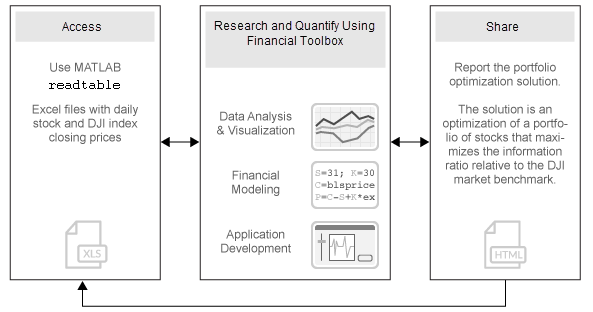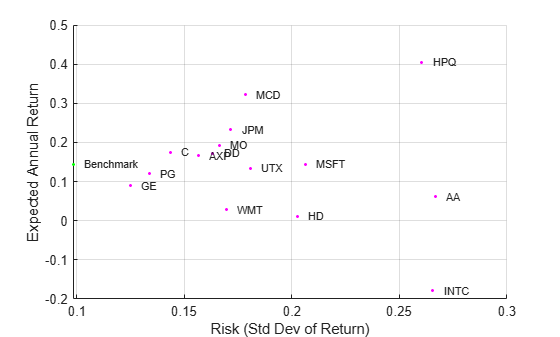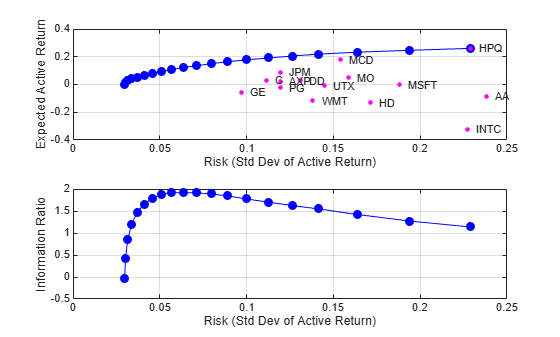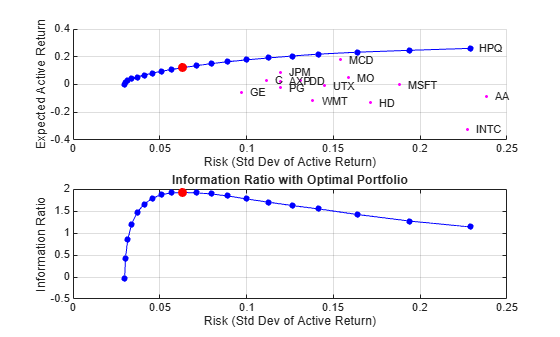Documentation

This is machine translation

Mouseover text to see original. Click the button below to return to the English version of the page.

Portfolio Optimization Against a Benchmark

This example shows how to perform portfolio optimization using the `Portfolio` object in Financial Toolbox™.

This example, in particular, demonstrates optimizing a portfolio to maximize the information ratio relative to a market benchmark. Specifically, financial data contained in a `table` is read into MATLAB® and visualizations (at both daily and annual levels) are performed. A Portfolio object is created with the market data using an active daily return for each asset. Using functions supporting a Portfolio object, the efficient frontier is calculated directly. Then, a customized optimization problem is solved to find the asset allocation with the maximized information ratio.Import Historical Data Using MATLAB®

Import historical prices for the asset universe and the Dow Jones Industrial Average (DJI) market benchmark. The data is imported into a `table` from a Microsoft® Excel® spreadsheet using the MATLAB® `readtable` function.

```data = readtable('dowPortfolio.xlsx'); head(data, 10)```
```ans=10×32 table Dates DJI AA AIG AXP BA C CAT DD DIS GE GM HD HON HPQ IBM INTC JNJ JPM KO MCD MMM MO MRK MSFT PFE PG T UTX VZ WMT XOM ___________ _____ _____ _____ _____ _____ _____ _____ _____ _____ _____ _____ _____ _____ _____ _____ _____ _____ _____ _____ _____ _____ _____ _____ _____ _____ _____ _____ _____ _____ _____ _____ 03-Jan-2006 10847 28.72 68.41 51.53 68.63 45.26 55.86 40.68 24.18 33.6 17.82 39.79 36.14 28.35 80.13 24.57 59.08 37.78 38.98 32.72 75.93 52.27 30.73 26.19 22.16 56.38 22.7 54.94 26.79 44.9 56.64 04-Jan-2006 10880 28.89 68.51 51.03 69.34 44.42 57.29 40.46 23.77 33.56 18.3 39.05 35.99 29.18 80.03 24.9 59.99 37.56 38.91 33.01 75.54 52.65 31.08 26.32 22.88 56.48 22.87 54.61 27.58 44.99 56.74 05-Jan-2006 10882 29.12 68.6 51.57 68.53 44.65 57.29 40.38 24.19 33.47 19.34 38.67 35.97 28.97 80.56 25.25 59.74 37.67 39.1 33.05 74.85 52.52 31.13 26.34 22.9 56.3 22.92 54.41 27.9 44.38 56.45 06-Jan-2006 10959 29.02 68.89 51.75 67.57 44.65 58.43 40.55 24.52 33.7 19.61 38.96 36.53 29.8 82.96 25.28 60.01 37.94 39.47 33.25 75.47 52.95 31.08 26.26 23.16 56.24 23.21 54.58 28.01 44.56 57.57 09-Jan-2006 11012 29.37 68.57 53.04 67.01 44.43 59.49 40.32 24.78 33.61 21.12 39.38 36.23 30.17 81.76 25.44 60.38 38.55 39.66 33.88 75.84 53.11 31.58 26.21 23.16 56.67 23.3 55.2 28.12 44.4 57.54 10-Jan-2006 11012 28.44 69.18 52.88 67.33 44.57 59.25 40.2 25.09 33.43 20.79 40.33 36.17 30.33 82.1 25.1 60.49 38.61 39.7 33.91 75.37 53.04 31.27 26.35 22.77 56.45 23.16 55.24 28.24 44.54 57.99 11-Jan-2006 11043 28.05 69.6 52.59 68.3 44.98 59.28 38.87 25.33 33.66 20.61 41.44 36.19 30.88 82.19 25.12 59.91 38.58 39.72 34.5 75.22 53.31 31.39 26.63 23.06 56.65 23.34 54.41 28.58 45.23 58.38 12-Jan-2006 10962 27.68 69.04 52.6 67.9 45.02 60.13 38.02 25.41 33.25 19.76 41.05 35.77 30.57 81.61 24.96 59.63 37.87 39.5 33.96 74.57 53.23 31.41 26.48 22.9 56.02 23.24 53.9 28.69 44.43 57.77 13-Jan-2006 10960 27.81 68.84 52.5 67.7 44.92 60.24 37.86 25.47 33.35 19.2 40.43 35.85 31.43 81.22 24.78 59.26 37.84 39.37 33.65 74.38 53.29 31.4 26.53 22.99 56.49 23.27 54.1 28.75 44.1 59.06 17-Jan-2006 10896 27.97 67.84 52.03 66.93 44.47 60.85 37.75 25.15 33.2 18.68 40.11 35.56 31.2 81.05 24.52 58.74 37.64 39.11 33.77 73.99 52.85 31.16 26.34 22.63 56.25 23.13 54.41 28.12 43.66 59.61 ```

Separate the asset names, asset prices, and DJI benchmark prices from the table. The visualization shows the evolution of all the asset prices normalized to start at unity, that is accumulative returns.

```dates = datenum(data.Dates); benchPrice = data.DJI; assetNames = data.Properties.VariableNames(3:2:end); % using half of the assets for display assetPrice = data(:,assetNames).Variables; assetP = assetPrice./assetPrice(1, :); benchmarkP = benchPrice / benchPrice(1); figure; plot(dates,assetP); hold on; plot(dates,benchmarkP,'LineWidth',3,'Color','k'); hold off; xlabel('Date'); ylabel('Normalized Price'); title('Normalized Asset Prices and Benchmark'); datetick('x'); grid on;```The bold line indicates the DJIA market benchmark.

Calculate the return series from the price series and compute the asset moments (historical returns and standard deviations). The visualization shows a scatter plot of the risk-return characteristics of all the assets and the DJI market benchmark.

```benchReturn = tick2ret(benchPrice); assetReturn = tick2ret(assetPrice); benchRetn = mean(benchReturn); benchRisk = std(benchReturn); assetRetn = mean(assetReturn); assetRisk = std(assetReturn);```

Calculate historical statistics and plot the annual risk-return. Note that the plot is at the annual level, therefore scaling is performed on the daily returns.

```scale = 252; assetRiskR = sqrt(scale) * assetRisk; benchRiskR = sqrt(scale) * benchRisk; assetReturnR = scale * assetRetn; benchReturnR = scale * benchRetn; figure; scatter(assetRiskR, assetReturnR, 6, 'm', 'Filled'); hold on scatter(benchRiskR, benchReturnR, 6, 'g', 'Filled'); for k = 1:length(assetNames) text(assetRiskR(k) + 0.005, assetReturnR(k), assetNames{k}, 'FontSize', 8); end text(benchRiskR + 0.005, benchReturnR, 'Benchmark', 'Fontsize', 8); hold off; xlabel('Risk (Std Dev of Return)'); ylabel('Expected Annual Return'); grid on;```Set Up a Portfolio Optimization

Set up a portfolio optimization problem by populating the object using `Portfolio`. Because the goal is to optimize portfolio allocation against a benchmark, the active return of each asset is computed and used in the `Portfolio` object. In this example, the expected returns and covariances of the assets in the portfolio are set to their historical values.

`p = Portfolio('AssetList',assetNames);`

Set up default portfolio constraints (all weights sum to `1`, no shorting, and 100% investment in risky assets).

`p = setDefaultConstraints(p);`

Add asset returns and covariance to the `Portfolio` object.

```activReturn = assetReturn - benchReturn; pAct = estimateAssetMoments(p,activReturn,'missingdata',false)```
```pAct = Portfolio with properties: BuyCost: [] SellCost: [] RiskFreeRate: [] AssetMean: [15x1 double] AssetCovar: [15x15 double] TrackingError: [] TrackingPort: [] Turnover: [] BuyTurnover: [] SellTurnover: [] Name: [] NumAssets: 15 AssetList: {1x15 cell} InitPort: [] AInequality: [] bInequality: [] AEquality: [] bEquality: [] LowerBound: [15x1 double] UpperBound: [] LowerBudget: 1 UpperBudget: 1 GroupMatrix: [] LowerGroup: [] UpperGroup: [] GroupA: [] GroupB: [] LowerRatio: [] UpperRatio: [] MinNumAssets: [] MaxNumAssets: [] BoundType: [15x1 categorical] ```

Compute the Efficient Frontier Using the `Portfolio` Object

Compute the mean-variance efficient frontier of 20 optimal portfolios. Visualize the frontier over the risk-return characteristics of the individual assets. Furthermore, calculate and visualize the information ratio for each portfolio along the frontier.

```pwgtAct = estimateFrontier(pAct, 20); % Estimate weights [portRiskAct, portRetnAct] = estimatePortMoments(pAct, pwgtAct); % Get risk and return % Extract asset moments & names [assetActRetnDaily, assetActCovarDaily] = getAssetMoments(pAct); assetActRiskDaily = sqrt(diag(assetActCovarDaily)); assetNames = pAct.AssetList; % Rescale assetActRiskAnnual = sqrt(scale) * assetActRiskDaily; portRiskAnnual = sqrt(scale) * portRiskAct; assetActRetnAnnual = scale * assetActRetnDaily; portRetnAnnual = scale * portRetnAct; figure; subplot(2,1,1); plot(portRiskAnnual, portRetnAnnual, 'bo-', 'MarkerFaceColor', 'b'); hold on; scatter(assetActRiskAnnual, assetActRetnAnnual, 12, 'm', 'Filled'); hold on; for k = 1:length(assetNames) text(assetActRiskAnnual(k) + 0.005, assetActRetnAnnual(k), assetNames{k}, 'FontSize', 8); end hold off; xlabel('Risk (Std Dev of Active Return)'); ylabel('Expected Active Return'); grid on; subplot(2,1,2); plot(portRiskAnnual, portRetnAnnual./portRiskAnnual, 'bo-', 'MarkerFaceColor', 'b'); xlabel('Risk (Std Dev of Active Return)'); ylabel('Information Ratio'); grid on;```Perform Information Ratio Maximization Using Optimization Toolbox™

Run a hybrid optimization to find the portfolio along the frontier with the maximum information ratio. The information ratio is the ratio of relative return to relative risk (known as "tracking error"). Whereas the Sharpe ratio looks at returns relative to a riskless asset, the information ratio is based on returns relative to a risky benchmark, in this case the DJI benchmark. This is done by running an optimization that finds the optimal return constraint for which the portfolio optimization problem returns the maximum information ratio portfolio. The portfolio optimization functions are called from an objective function `infoRatioTargetReturn` that is optimized by the Optimization Toolbox™ function `fminbnd`. The local function `infoRatioTargetReturn` calculates a minimum (active) risk portfolio given a target active return.

The `infoRatioTargetReturn` local function is called as an objective function in an optimization routine (`fminbnd`) that seeks to find the target return that maximizes the information ratio and minimizes a negative information ratio.

```objFun = @(targetReturn) -infoRatioTargetReturn(targetReturn,pAct); options = optimset('TolX',1.0e-8); [optPortRetn, ~, exitflag] = fminbnd(objFun,0,max(portRetnAct),options);```

Get weights, information ratio, and risk return for the optimal portfolio.

```[optInfoRatio,optWts] = infoRatioTargetReturn(optPortRetn,pAct); optPortRisk = estimatePortRisk(pAct,optWts) ```
```optPortRisk = 0.0040 ```

Plot the Optimal Portfolio

Verify that the portfolio found is indeed the maximum information-ratio portfolio.

```% Rescale optPortRiskAnnual = sqrt(scale) * optPortRisk; optPortReturnAnnual = scale * optPortRetn; figure; subplot(2,1,1); scatter(assetActRiskAnnual, assetActRetnAnnual, 6, 'm', 'Filled'); hold on for k = 1:length(assetNames) text(assetActRiskAnnual(k) + 0.005,assetActRetnAnnual(k),assetNames{k},'FontSize',8); end plot(portRiskAnnual,portRetnAnnual,'bo-','MarkerSize',4,'MarkerFaceColor','b'); plot(optPortRiskAnnual,optPortReturnAnnual,'ro-','MarkerFaceColor','r'); hold off; xlabel('Risk (Std Dev of Active Return)'); ylabel('Expected Active Return'); grid on; subplot(2,1,2); plot(portRiskAnnual,portRetnAnnual./portRiskAnnual,'bo-','MarkerSize',4,'MarkerFaceColor','b'); hold on plot(optPortRiskAnnual,optPortReturnAnnual./optPortRiskAnnual,'ro-','MarkerFaceColor','r'); hold off; xlabel('Risk (Std Dev of Active Return)'); ylabel('Information Ratio'); title('Information Ratio with Optimal Portfolio'); grid on;```Display the Portfolio Optimization Solution

Display the portfolio optimization solution.

```assetIndx = optWts > .001; results = table(assetNames(assetIndx)', optWts(assetIndx)*100, 'VariableNames',{'Asset', 'Weight'}); disp('Maximum Information Ratio Portfolio:');```
```Maximum Information Ratio Portfolio: ```
`disp(results);`
``` Asset Weight ______ ______ 'AA' 1.539 'AXP' 0.3555 'C' 9.6533 'DD' 4.0684 'HPQ' 17.698 'JPM' 21.565 'MCD' 26.736 'MO' 13.648 'MSFT' 2.6858 'UTX' 2.0509 ```
`fprintf('Max. Info Ratio portfolio has expected active return %0.2f%%\n', optPortRetn*25200);`
```Max. Info Ratio portfolio has expected active return 12.14% ```
`fprintf('Max. Info Ratio portfolio has expected tracking error of %0.2f%%\n', optPortRisk*sqrt(252)*100);`
```Max. Info Ratio portfolio has expected tracking error of 6.32% ```

Local Function

```function [infoRatio,wts] = infoRatioTargetReturn(targetReturn,portObj) % Calculate information ratio for a target-return portfolio along the % efficient frontier wts = estimateFrontierByReturn(portObj,targetReturn); portRiskAct = estimatePortRisk(portObj,wts); infoRatio = targetReturn/portRiskAct; end```